Subsections

# Inhomogeneous Systems

## Problem

In inhomogeneous systems G depends on the value of x in all points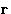. G is a functional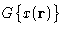--it depends on a function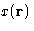.

Analogy with multi variable case:
we have variables,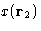,..., and want to minimize G.
Idea:
Introduce normal coordinates that diagonalize G
Trick:
Use Fourier transform--it leads to normal coordinates in linear problems

## Landau Hamiltonian and Normal Coordinates

For inhomogeneous system the free energy is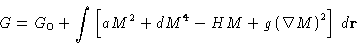Assumptions and simplifications:
1.
We are above critical point, so a>0 (for negative a we expand around M0)
2.
We neglect the term dM4, This is the most important approximation!!!

Then we make Fourier transform: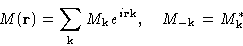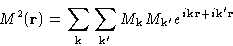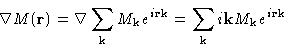and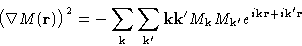Term with external field: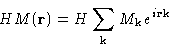Mathematical result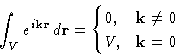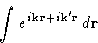This is non-zero only if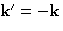. Then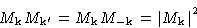and we obtain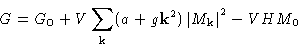We represented free energy as a sum over Fourier components--this means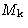are normal coordinates!

## Fluctuations in Fourier Space. Scattering

We immediately obtain for fluctuations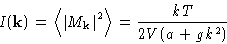This is exactly the function measured in scattering experiments!

What happens near critical points? Hereis small.

1.
Short wavelength fluctuations do not depend on the temperature: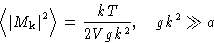2.
Long wavelength fluctuations grow: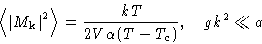3.
The characteristic wavelength tends to infinity: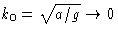This is called critical opalescence

## Correlation in Real Space. Ornstein-Zernicke Function

The function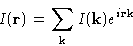Is called correlation function. It is just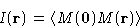Integrating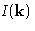, we obtain: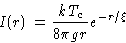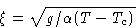This function is called Ornstein-Zernicke function.

What happens at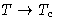? Correlation radius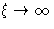1.
At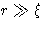we have exponential decay with distance
2.
At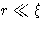correlations decay as 1/r and are independent of the temperature!

Above we obtained the formula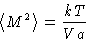This works in thermodynamic limit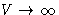. In other words. Near Tc this condition becomes very stringent!

## Ginsburg Number

Landau theory works if fluctuations are small. Average fluctuation in the volume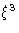is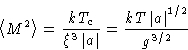Comparing this to the equilibrium value below critical point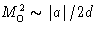, we obtain that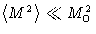if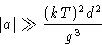or, since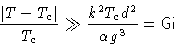(4)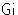is Ginsburg number.

Landau theory works, if we are both:

1.
Close to critical point, so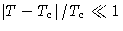(we used expansion!)
2.
Still not close enough, so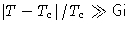.

Why does Landau theory work well for polymers? Coefficient g does not depend on molecular weight N, butand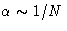. We obtain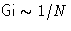, i.e. Ginsburg number is small.Next: Universality and Scaling Invariance Up: Fluctuations in Inhomogeneous Systems. Previous: Fluctuations in Homogeneous Systems

© 1997 Boris Veytsman and Michael Kotelyanskii
Tue Oct 28 22:10:23 EST 1997